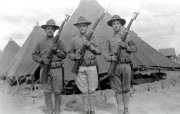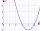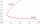# Trio

56 children lined up in groups of three. How many children did not create a trio?

Result

n =  2

#### Solution:

$n = 56 \mod \ 3 = 2$Our examples were largely sent or created by pupils and students themselves. Therefore, we would be pleased if you could send us any errors you found, spelling mistakes, or rephasing the example. Thank you!

Leave us a comment of this math problem and its solution (i.e. if it is still somewhat unclear...):Be the first to comment!## Next similar math problems:

1. Year 2018The product of the three positive numbers is 2018. What are the numbers?
2. Collection of stampsJano, Rado, and Fero have created a collection of stamps in a ratio of 5: 6: 9. Two of them had 429 stamps together. How many stamps did their shared collection have?
3. DozenWhat is the product of 26 and 5? Write the answer in Arabic numeral. Add up the digits. How many of this is in a dozen? Divide #114 by this
4. Evaluate - order of opsEvaluate the expression: 32+2[5×(24-6)]-48÷24 Pay attention to the order of operation including integers
5. Expression plus minusEvaluate expression: (-1)2 . 12 – 6 : 3 + (-3) . (-2) + 22 – (-3) . 2
6. Evaluate 5Evaluate expression x2−7x+12x−4 when x=−1In about 12 hours in North Dakota the temperature rose from -33 degrees farenheit to 50 degrees farenheit. By how much did the temperature change?
8. The temperatureThe temperature at 1:00 was 10 F. Between 1:00 and 2:00, the temperature dropped 15F. Between 2:00 and 3:00, the temperature rose 3F. What is the temperature at 3:00?
9. SimplifySimplify expression - which expression is equivalent to: 3(m + 2) − 4(2m − 9)
10. Progression12, 60, -300,1500 need next 2 numbers of pattern
11. Evaluate expression 2Evaluate expression with negatives: (-3)+4+(-8)+(-6)+4+(-1)
12. Degrees 2The temperature was 3°F and falls four degrees Fahrenheit. What is actually temperature?
13. IntegerFind the integer whose distance on the numerical axis from number 1 is two times smaller as the distance from number 6.
14. Expression 6Evaluate expression: -6-2(4-8)-9
15. Two integersTwo integers, a and b, have a product of 36. What is the least possible sum of a and b?
16. The differenceThe difference of two numbers is 1375. If their exact quotient is 12. Find the two numbers
17. EquatiomSolve equation with negatives: X/(-5) + 2 = -9